Solving Pair of Linear Inequalities

Chapter 5 Class 11 Linear Inequalities
Serial order wise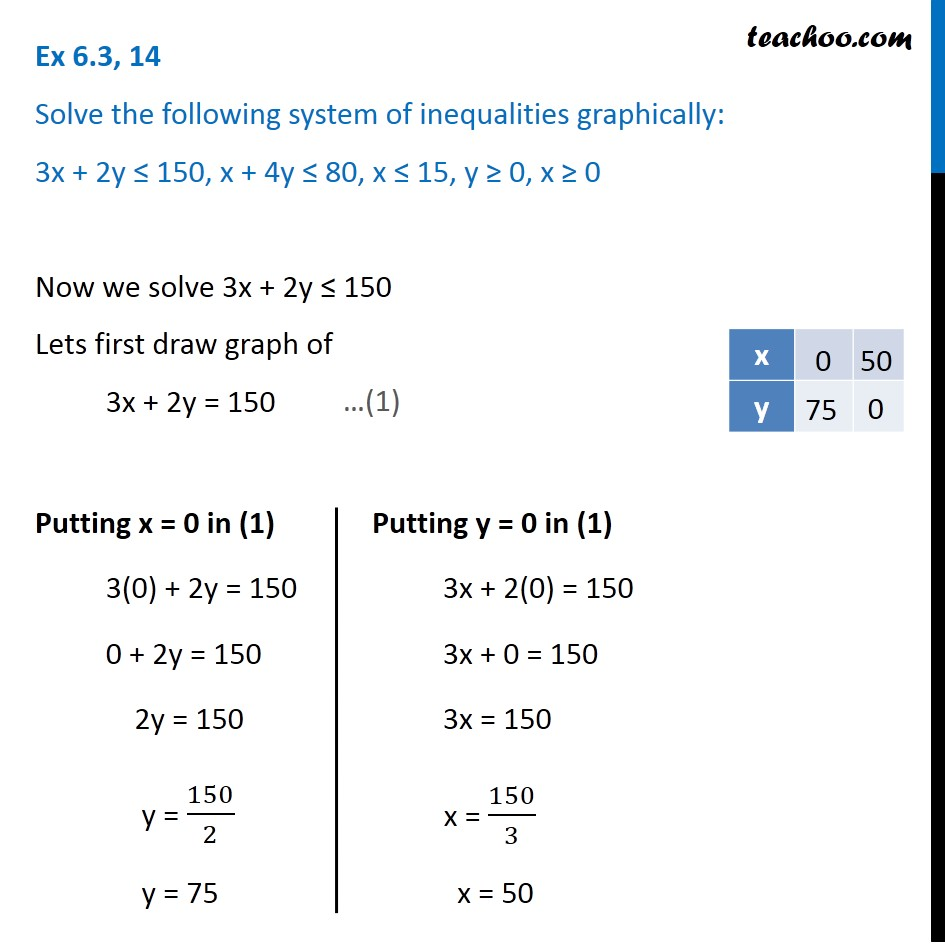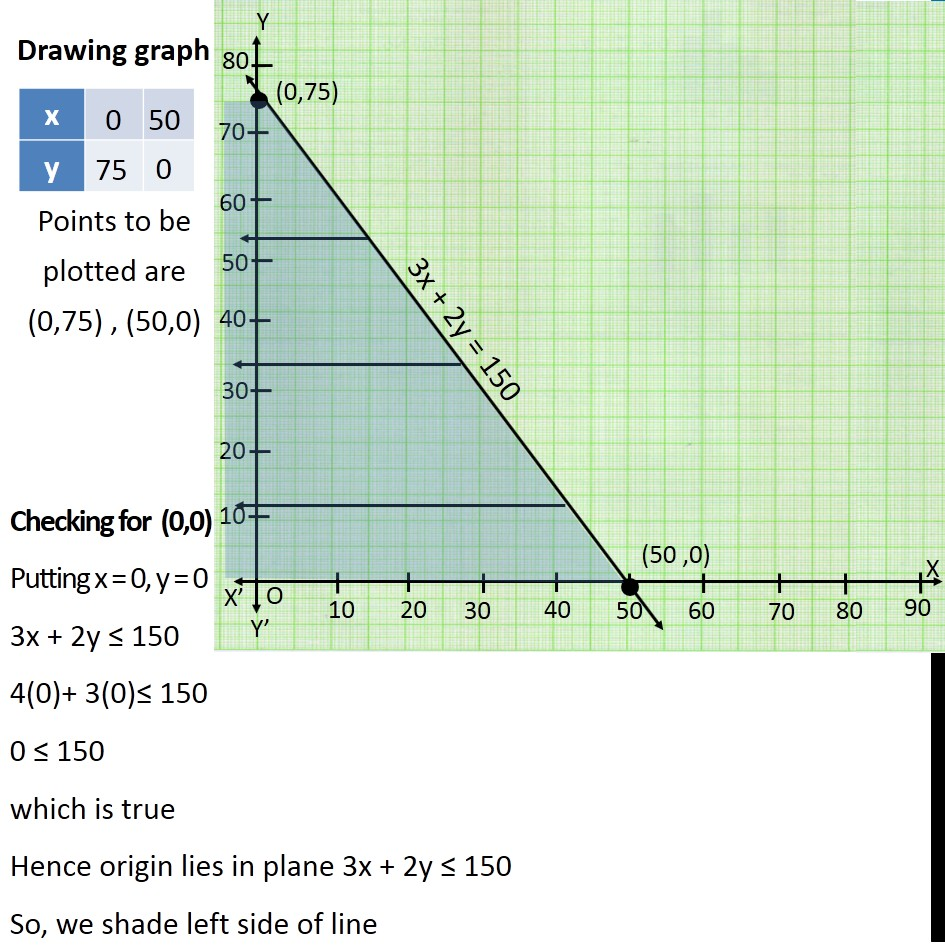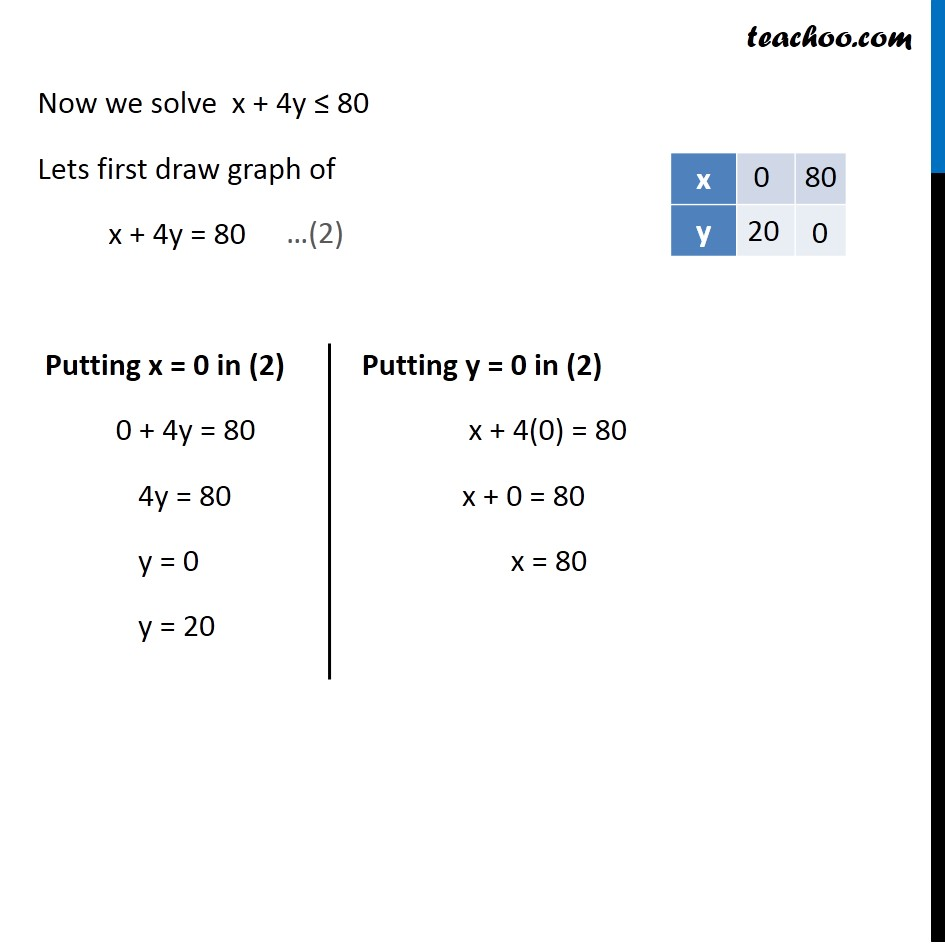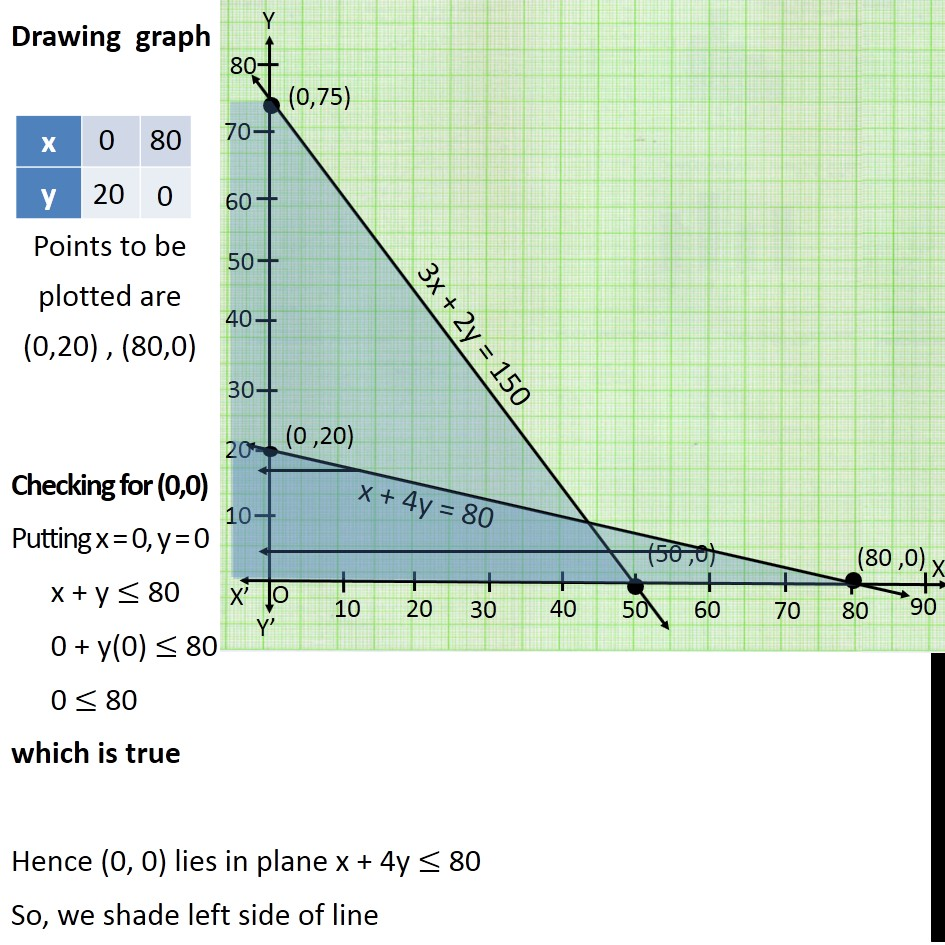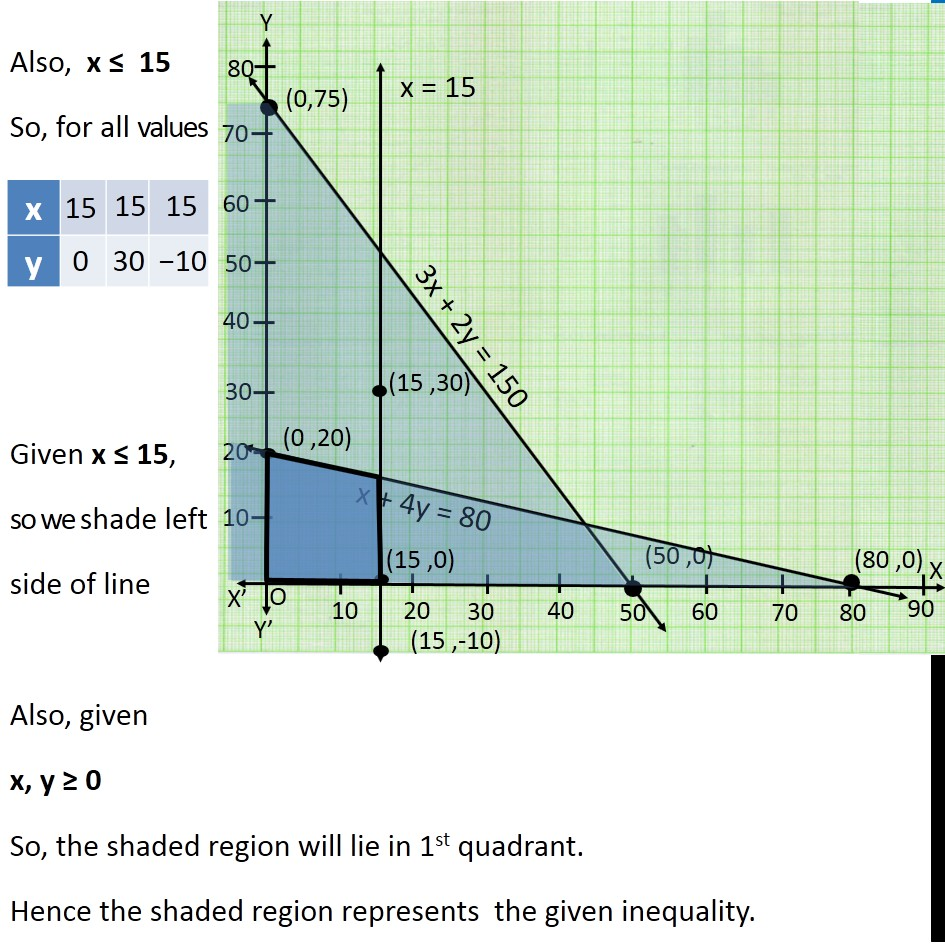Learn in your speed, with individual attention - Teachoo Maths 1-on-1 Class

### Transcript

Question 14 Solve the following system of inequalities graphically: 3x + 2y ≤ 150, x + 4y ≤ 80, x ≤ 15, y ≥ 0, x ≥ 0 Now we solve 3x + 2y ≤ 150 Lets first draw graph of 3x + 2y = 150 Putting x = 0 in (1) 3(0) + 2y = 150 0 + 2y = 150 2y = 150 y = 150/2 y = 75 Putting y = 0 in (1) 3x + 2(0) = 150 3x + 0 = 150 3x = 150 x = 150/3 x = 50 Points to be plotted are (0,75) , (50,0) Drawing graph Checking for (0,0) Putting x = 0, y = 0 3x + 2y ≤ 150 4(0)+ 3(0)≤ 150 0 ≤ 150 which is true Hence origin lies in plane 3x + 2y ≤ 150 So, we shade left side of line Now we solve x + 4y ≤ 80 Lets first draw graph of x + 4y = 80 Putting x = 0 in (2) 0 + 4y = 80 4y = 80 y = 0a y = 20 Putting y = 0 in (2) x + 4(0) = 80 x + 0 = 80 x = 80 Points to be plotted are (0,20) , (80,0) Drawing graph Checking for (0,0) Putting x = 0, y = 0 x + y ≤ 80 0 + y(0) ≤ 80 0 ≤ 80 which is true Hence (0, 0) lies in plane x + 4y ≤ 80 So, we shade left side of line Also, x ≤ 15 So, for all values of y, x = 15 Given x ≤ 15, so we shade left side of line Also, given x, y ≥ 0 So, the shaded region will lie in 1st quadrant. Hence the shaded region represents the given inequality.